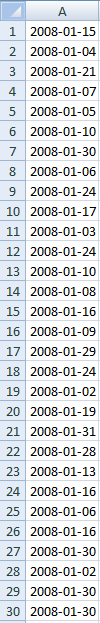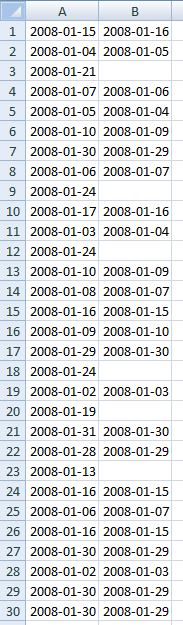Author: Oscar Cronquist Article last updated on October 05, 2018

Question: How to identify two consecutive dates in a list?Array formula in cell B1:

=IFERROR(LARGE(IF((A1+1)=\$A\$1:\$A\$30,A1+1,""),1),"")

How to enter an array formula

1. Select cell B1
2. Copy above formula (Ctrl + c)
3. Press with left mouse button on in formula bar4. Paste formula (Ctrl + v)
5. Press and hold CTRL + SHIFT
6. Press Enter
7. Release all keys

### Explaining array formula in cell B1

Step 1 - Check if next consecutive date is in cell range

IF((A1+1)=\$A\$1:\$A\$30,A1+1,"")

becomes

IF((39462+1)={39462; 39451; 39468; 39454; 39452; 39457; 39477; 39453; 39471; 39464; 39450; 39471; 39457; 39455; 39463; 39456; 39476; 39471; 39449; 39466; 39478; 39475; 39460; 39463; 39453; 39463; 39477; 39449; 39477; 39477},39462+1,"")

and returns

{""; ""; ""; ""; ""; ""; ""; ""; ""; ""; ""; ""; ""; ""; 39463; ""; ""; ""; ""; ""; ""; ""; ""; 39463; ""; 39463; ""; ""; ""; ""}

Step 2 - Return the largest value

LARGE(IF((A1+1)=\$A\$1:\$A\$30,A1+1,""),1)

becomes

LARGE(IF({""; ""; ""; ""; ""; ""; ""; ""; ""; ""; ""; ""; ""; ""; 39463; ""; ""; ""; ""; ""; ""; ""; ""; 39463; ""; 39463; ""; ""; ""; ""},1)

and returns 39463 (2008-01-16) in cell B1.

Step 3 - Remove errors

If the LARGE function returns an error the IFERROR function removes that error. That happens every time there isn´t a following consecutive date.

### Get excel *.xlsx file

identify-2-consecutive-dates-in-a-list-in-excelv3.xlsx

### Functions in this post

IF(logical_test, [value_if_true], [value_if_false])
Checks whether a condition is met, and returns one value if TRUE, and another value if FALSE

LARGE(arrayk)
Returns the k-th largest value in a data set.